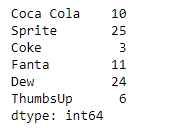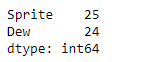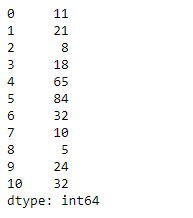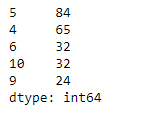GeeksforGeeks App
Open AppBrowser
Continue

# Python | Pandas Series.nlargest()

Pandas series is a One-dimensional ndarray with axis labels. The labels need not be unique but must be a hashable type. The object supports both integer- and label-based indexing and provides a host of methods for performing operations involving the index.

Pandas` Series.nlargest()` function return the n largest element from the underlying data in the given series object.

Syntax: Series.nlargest(n=5, keep=’first’)

Parameter :
n : Return this many descending sorted values.
keep : {‘first’, ‘last’, ‘all’}, default ‘first’

Returns : Series

Example #1: Use `Series.nlargest()` function to return the first n largest element from the given series object.

 `# importing pandas as pd``import` `pandas as pd`` ` `# Creating the Series``sr ``=` `pd.Series([``10``, ``25``, ``3``, ``11``, ``24``, ``6``])`` ` `# Create the Index``index_ ``=` `[``'Coca Cola'``, ``'Sprite'``, ``'Coke'``, ``'Fanta'``, ``'Dew'``, ``'ThumbsUp'``]`` ` `# set the index``sr.index ``=` `index_`` ` `# Print the series``print``(sr)`

Output :Now we will use `Series.nlargest()` function to find the first 2 largest value in the given series object.

 `# return the first 2 of the largest``# element``result ``=` `sr.nlargest(n ``=` `2``)`` ` `# Print the result``print``(result)`

Output :As we can see in the output, the `Series.nlargest()` function has successfully returned the first 2 largest value in the given series object.

Example #2: Use `Series.nlargest()` function to return the first n largest element from the given series object.

 `# importing pandas as pd``import` `pandas as pd`` ` `# Creating the Series``sr ``=` `pd.Series([``11``, ``21``, ``8``, ``18``, ``65``, ``84``, ``32``, ``10``, ``5``, ``24``, ``32``])`` ` `# Print the series``print``(sr)`

Output :Now we will use `Series.nlargest()` function to find the first 5 largest value in the given series object.

 `# return the first 5 of the largest``# element``result ``=` `sr.nlargest(n ``=` `5``)`` ` `# Print the result``print``(result)`

Output :As we can see in the output, the `Series.nlargest()` function has successfully returned the first 5 largest value in the given series object.

My Personal Notes arrow_drop_up